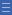## Featured resource

Home > Topdrawer > Mental computation > Activities > Investigating patterns

# Investigating patterns

Investigating patterns

In this activity, students construct a rectangular array for a given number from which they will generate and record the multiplication facts and the list of factors.

When students investigate patterns on a 1–100 chart or on a multiplication fact grid, it assists their understanding of properties (e.g. the identity and commutative properties).

Display the multiplication fact grid and give the students a few minutes to discuss in pairs any patterns they notice.

List students' ideas on the board (e.g. the first row and column in the unshaded section are all zeros, the second row and column are counting by ones, each column has a corresponding row that is the same).

Ask: Why do you think the numbers in the first row and column all end is zero? What does this mean?

Encourage students to use models and everyday examples to develop a convincing reason.

Explore the second column and row (counting by ones), and the square numbers (1, 4, 9, 16, 25 etc.) in a similar manner.

Some teaching tips about possible patterns can be seen in the following slide show.Year 3: Recall multiplication facts of two, three, five and ten and related division facts Infobox 3Year 4: Recall multiplication facts up to 10 x 10 and related division facts Infobox 3